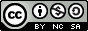# Why is this so Terrible?

Elements of Functional Programming for React.js Developers

2020-01-16

This presentation is also available at

Source code with most examples:Made with love using Pandoc, RevealJS and Neovim.
Thanks for all the contributors for these awesome tools.

How can you trust your data
when it has its own mind?

# Essence of Object Oriented Programming

The data, state and the computation are coupled together:

``````const ar = [ 1, 2, 3 ]
console.log(ar.pop())
console.log(ar)``````

Each object is a little actor with its own state.

It exposes various methods
via which other actors can communicate with it.

They are all one big, happy family
that interacts with one another.

Sounds like a powerful idea.

# The Good

There are some benefits of OOP

e.g. it is easy to implement stateful computation.

``````const stateful = {
count: 0,
poke() {
this.count = this.count + 1
if (this.count > 3) {
console.log("**** you!")
} else {
console.log("a! ".repeat(this.count))
}
}
}

setInterval(() => stateful.poke(), 500)``````

But

many actors, each with its own state

sounds complex.

And it is!

You have to keep track of implicit context

``````"use strict"

const speaker = {
introduce() {
console.log(`Hello, I'm \${this.name}!`)
}
}
speaker.introduce()
setTimeout(speaker.introduce, 500)``````

When you implement a function

(method is just a function attached to an object)

you don’t know what `this` will be.

So `this` is like an implicit argument.

It will only be known at the time of calling the function.

``````"use strict"

const fn = function() { console.log(this) }
const ob = { fn, fatfn:  () => fn() }

fn(4)
fn.call(3)
fn.apply(2)
fn.bind(1)
ob.fn(0)
ob.fatfn(-1)``````

# The Ugly

Even worse, your data starts having brain of its own:

``````"use strict"

const ar = [ 1, 2, 3, 4 ]``````
``ar.reduce = () => "Buhahaha 🥳"``
``console.log(ar.reduce((e, memo) => e * memo, 1))``

Hint: It will print “Buhahaha 🥳”

And it may be up to no good

``````"use strict"

const a = {
i: 1,
valueOf() { return this.i++; }
}``````
``````if ( (a == 1) && (a == 2) && (a == 3) ) {
console.log("I'll be damned.")
}``````

Seriously, who needs this in their language?

# What’s the alternative

``[ 1, 2, 3 ].map((e) => e + 1);``

why not

``map((e) => e + 1, [ 1, 2, 3 ])``

?

``[ 1, 2, 3 ].length;``

why not

``length([ 1, 2, 3 ])``

?

# The Essence of Functional Programming

### Composition.

Things are simple and you can compose them

A data is just a data: `a`

A function is just a pure function: `a -> b`

Functions don’t have state nor side - effects.

You can compose data:

``````const a = 1
const b = 2
const composed = { a, b }``````

You can compose functions:

``````"use strict"

const increment = function (number) { return number + 1 }
const double = function (number) { return number * 2 }

const compute = function (number) {
return increment(double(number))
}

console.log(compute(3))``````

Ugly?

``````const compute = function (number) {
return increment(double(number))
}``````

``````const flow = require("lodash/fp/flow")

const compute_too = flow(double, increment)
``````

Or even this:

``flow(compute_too, console.log)(3)``

With this setup:

``````const increment = function (number) { return number + 1 }
const double = function (number) { return number * 2 }
const compute = flow(double, increment)``````

All of these are equivalent:

``````console.log(increment(double(3)))
console.log(flow( double, increment )(3))
console.log(compute(3))
flow( double, increment, console.log )(3)
flow( compute, console.log )(3)``````

But what if the function I want to compose takes some additional arguments?

``function divideBy (a, b) { return b / a }``

The lambda way:

make your self a new function

``````const divideBy = function (a, b) { return b / a }

const compute = flow(
double,
increment,
(number) => divideBy(3, number)
)

console.log(compute(4))``````

The partial way:

``````const compute = flow(
double,
increment,
partial(divideBy, )
)

console.log(compute(4))``````

The Indian cuisine way

``````const divideBy = curry(function (a, b) { return b / a })

const compute = flow(
double,
increment,
divideBy(3)
)

console.log(compute(4))``````

Just kidding. It’s a way of Haskell Brooks Curry.

Using partial application and composition you can model any computation, no matter how complex.

And it will almost always be easier to reason about than OOP, because no state means less complexity.

# Simplicity

Predictible behaviour, simple rules of composition and partial application.

What follows is that concerns are usually separated

### data | computation | state | effects

You can mix them if you want to, but by default they are separated.

# But I need my state!

Sure. Sometimes you do. But ask yourself how often?

And if you really do, be explicit about it.

If you are working with React, use Redux.

(or whatever else is all the hype this week)

If you need effects (e.g. networking), use Thunks.

For presentation use functional components.

# Educate Yourself

• How to do FP in JS